The combination of Errors:

• Error of A Sum or A Difference
• Error of A Product or A Quotient
• Error in case of a measured quantity raised to a power

### Error of A Sum or A Difference:

Rule: When two quantities are added or subtracted, the absolute error in the final result is the sum of the absolute errors in the individual quantities.

Explanation: Let two physical quantities A and B have measured values A ± ΔA, B ± ΔB respectively, where ΔA and ΔB are their absolute errors.

We wish to find the error ΔZ in the sum

Z = A + B.

Z ± ΔZ = (A ± ΔA) + (B ± ΔB).

The maximum possible error in Z

ΔZ = ΔA + ΔB

For the difference Z = A – B, we have

Z ± Δ Z = (A ± ΔA) (B ± ΔB)
= (A – B) ± ΔA ± ΔB
or,  ± ΔZ = ± ΔA ± ΔB

The maximum value of the error ΔZ is again ΔA + ΔB.

### Error of A Product or A Quotient:

Rule: When two quantities are multiplied or divided, the relative error in the result is the sum of the relative errors in the multipliers.

Explanation: Let Z = AB and the measured values of A and B are A ± ΔA and B ± ΔB. Then

Z ± ΔZ = (A ± ΔA) (B ± ΔB)

Z ± ΔZ = AB ± B ΔA ± A ΔB ± ΔA ΔB

Dividing LHS by Z and RHS by AB we have,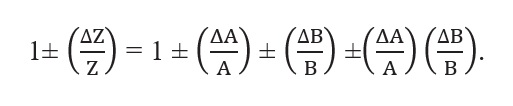Since ΔA and ΔB are small, we shall ignore their product.

Hence the maximum relative error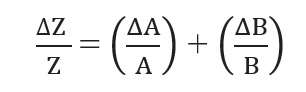You can easily verify that this is true for division also.

### Error in case of a measured quantity raised to a power:

Rule: The relative error in a physical quantity raised to the power k is the k times the relative error in the individual quantity.

Explanation: Suppose Z = A2,

Then,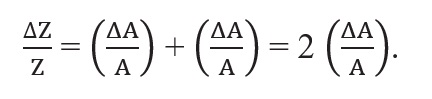Hence, the relative error in A2 is two times the error in A.

In general, if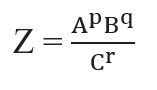Then,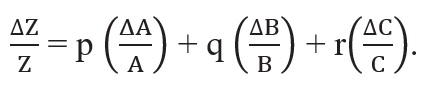The physical quantity Z with absolute error ∆Z is then expressed as, Z ± ∆Z